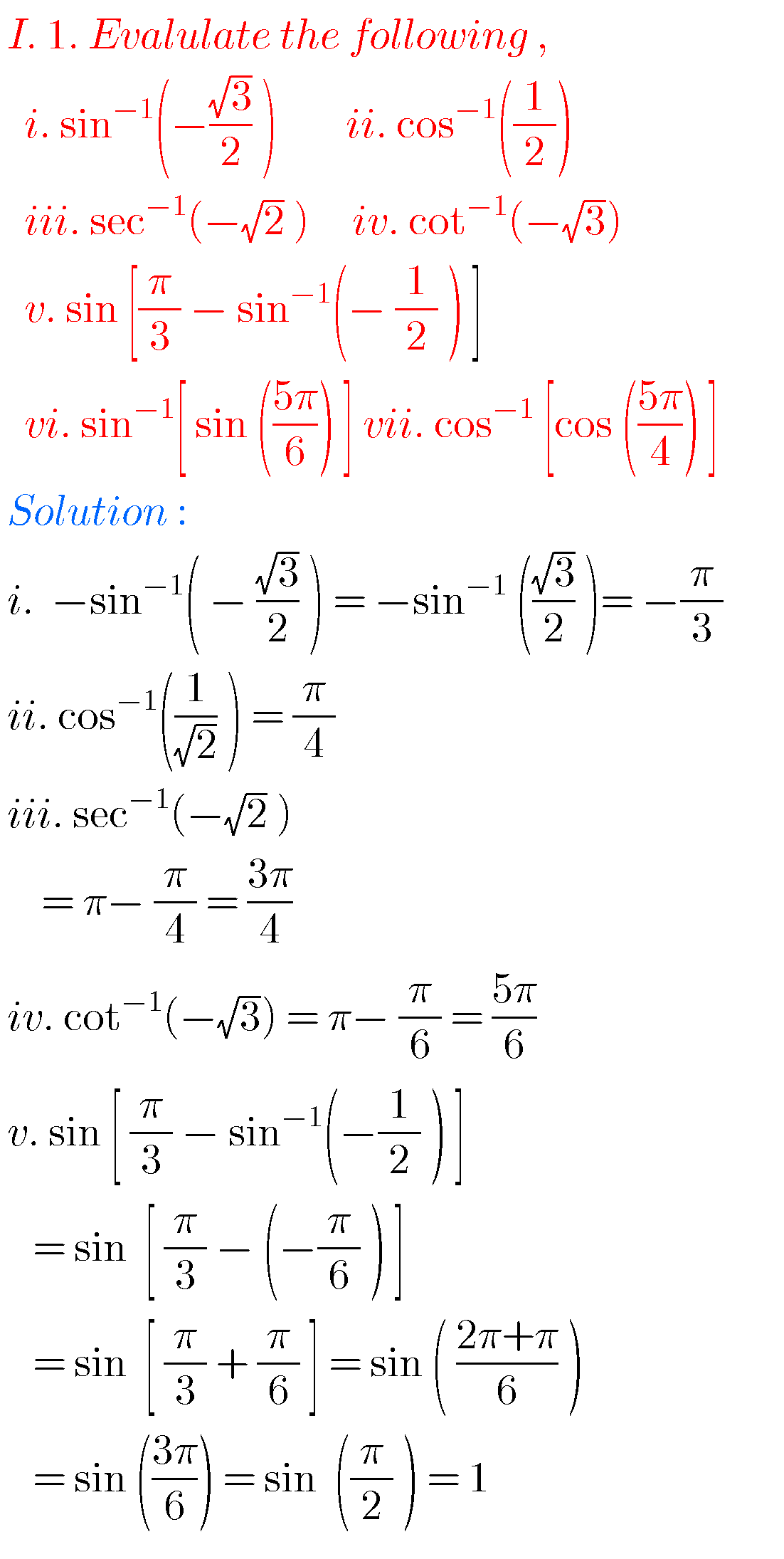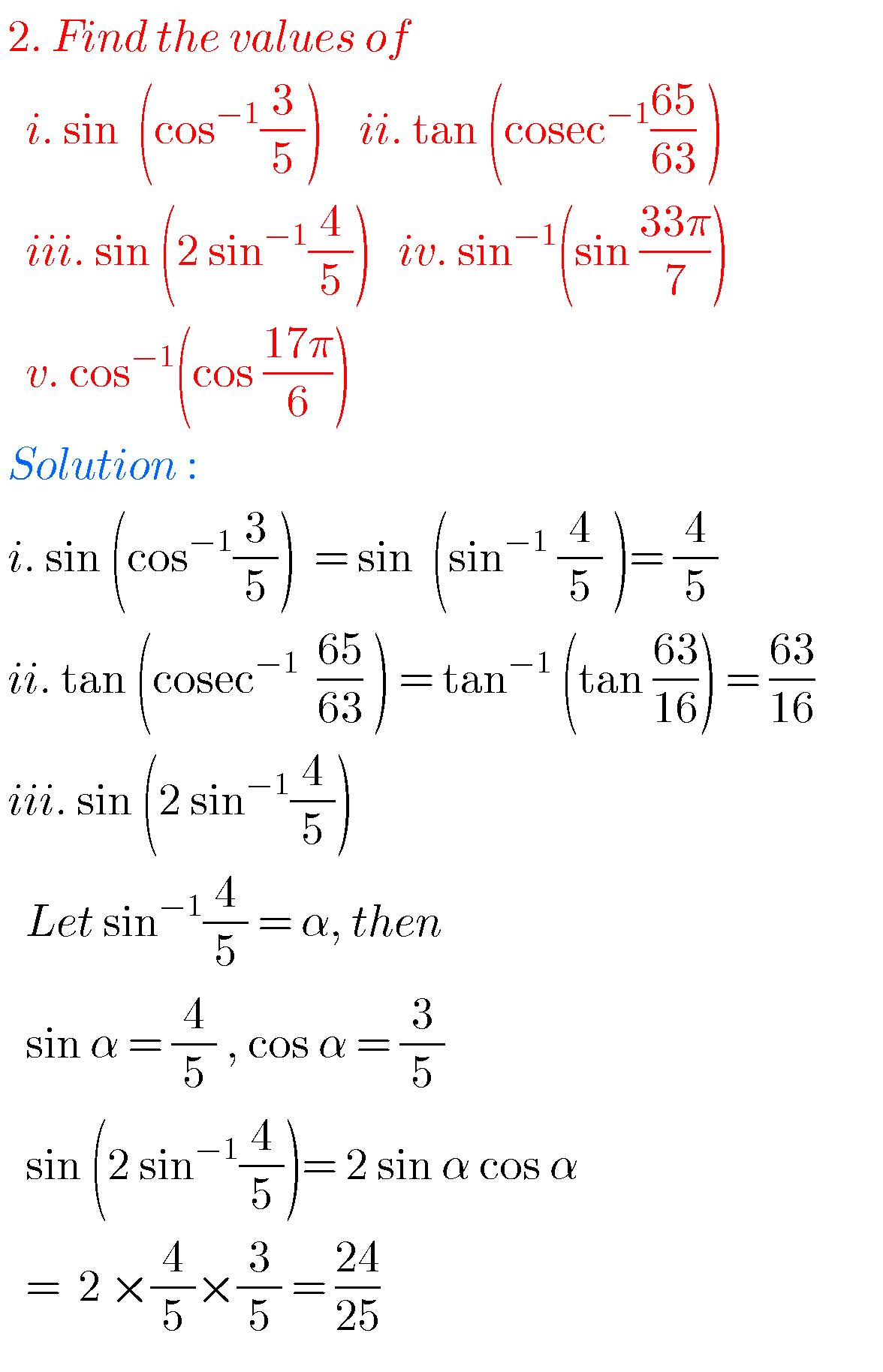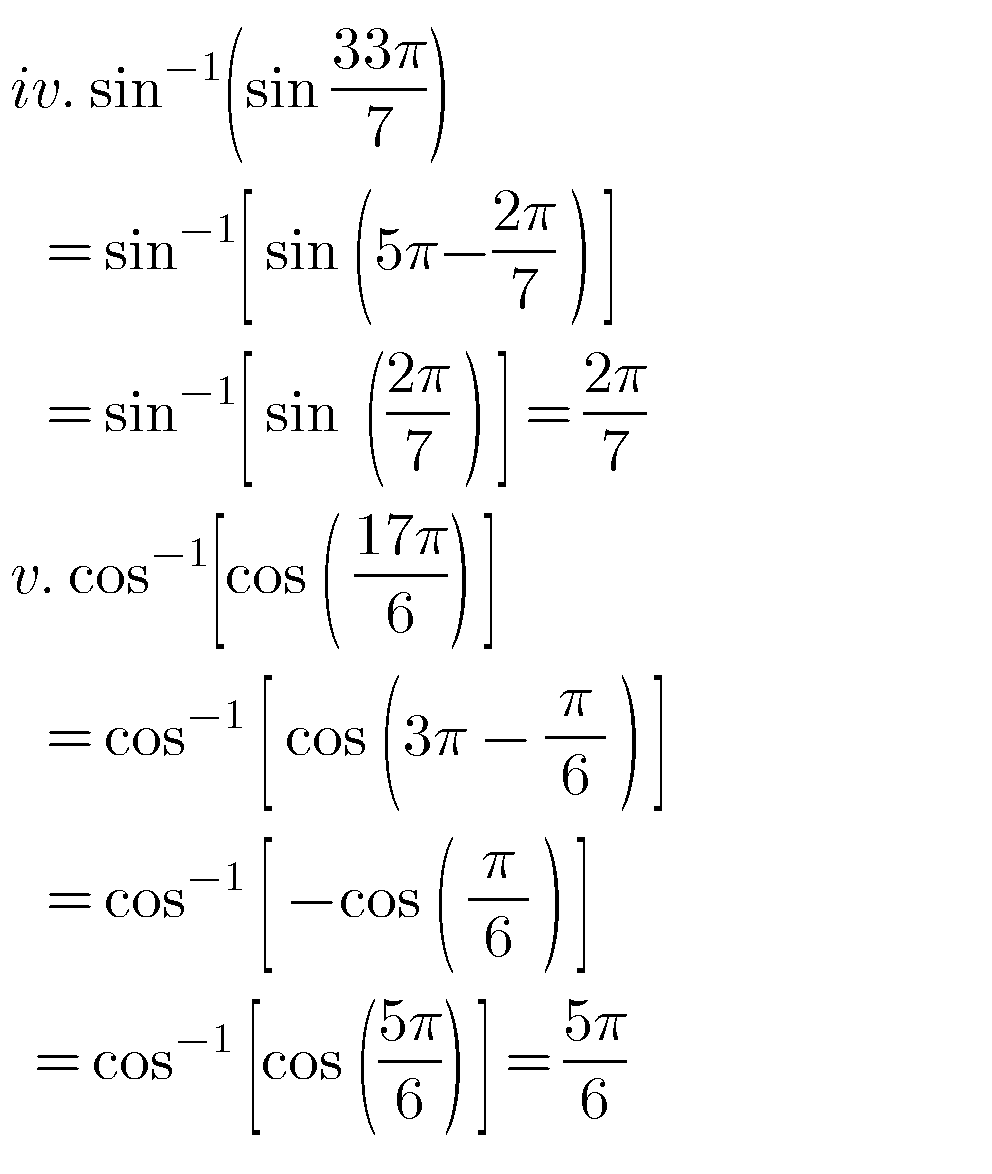# INVERSE TRIGONOMETRIC FUNCTIONS, INTERMEDIATE FIRST YEAR MATHEMATICS 1A CHAPTER 8 PROBLEMS WITH SOLUTIONS

Mathematics intermediate first year 1A solutions for some problems.

These solutions are very easy to understand.

Study the textbook lesson Inverse Trigonometric Functions very well.

Observe the example problems and solutions given in the textbook.

Text book exercise solutions links are also given.

Inverse Trigonometric Equations

Exercise 8(a)

You can also see

Inter Maths 1A textbook solutions

Inter Maths 1B textbook solutions

Inter Maths IIA textbook solutions

Inter Maths IIB textbook solutions

Nios solutions for maths 311 book 1 Sets 1.1

M

# JUNIOR INTER MATHS SOLUTIONS FOR TRIGONOMETRY

### Inverse trigonometric functions solutions3ii

Iii2 i3

M

## JUNIOR INTER MATHS SOLUTIONS FOR TRIGONOMETRY

Note : Observe the solutions of inverse trigonometric functions and try them in your own methods.

You can see the solutions for junior inter maths 1A

3. Matrices

Ncert solutions for maths class 6 th some chapters

Ncert solutions for maths class 7 th some chapters

Ncert solutions for maths class 8 th some chapters

Mathematics solutions for some chapters for some classes

Ncert solutions for class 8 chapter 12

Inter maths trigonometry solutions

Maths solutions class 10

### 2 thoughts on “Inverse Trigonometric Functions,Intermediate first year Mathematics 1A chapter 8 problems with solutions,junior inter maths solutions for Trigonometry”

1.I required short and very short answers from this inverse trignometric functions chapter

2.I need all long answers from inverse trigonometric functions